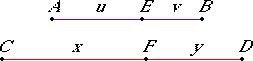# Definition 14

A ratio taken jointly means taking the antecedent together with the consequent as one in relation to the consequent by itself.

# Definition 15

A ratio taken separately means taking the excess by which the antecedent exceeds the consequent in relation to the consequent by itself.

# Definition 16

Conversion of a ratio means taking the antecedent in relation to the excess by which the antecedent exceeds the consequent.

## Guide

 Taking jointly the ratio u : v yields the ratio (u + v):v. Taking separately the ratio (u + v):v returns the ratio u : v. Taking the ratio (u + v):v in conversion yields the ratio (u + v):u. These conversions are only important when the ratios are in proportions. The following three proportions are shown to be equivalent in propositions V.17 and V.18. 1. (u + v) : v = (x + y) : y. 2. (u + v) : u = (x + y) : x. 3. u : v = x : y.Proposition V.17 and V.18 show proportions 1 and 3 are equivalent. That means proportion 2 and the inverse of 3, v : u = y : x, are also equivalent. And of course, 3 and its inverse are equivalent, so all three proportions are equivalent. Furthermore, when all the magnitudes are of the same kind, then the alternate proportions are also equivalent by V.16 making six equivalent statements. 4. (u + v) : (x + y) = v : y. 5. (u + v) : (x + y) = u : x 6. u : x = v : y Proposition V.19 goes on to say that 4 implies 5, and its corollary says 1 implies 2. Heath translates “taken jointly,” “taken separately,” and “in conversion” by the Latin words componendo, separando, and convertendo, respectively.